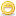CodeGuru Home VC++ / MFC / C++ .NET / C# Visual Basic VB Forums Developer.com

# Thread: anyone can help me with this?

1.Junior MemberJoin Date
Sep 2018
Posts
2

##anyone can help me with this?

Write a program that asks the user to enter three real numbers. if all three numbers are positives, print the sum else if one number is negative print the product of the two positive numbers. you will then ask the user to enter two real numbers. if both numbers are negative print the quotient, otherwise terminate the program. use fixed mode and format the output, in other words, specify the width and the number of digits meaningful variable names and informative prompts

1
2
3
4
5
6
7
8
9
10
11
12
13
14
15
16
17
18
19
20
21
22
23
24
25
26
27
28
29
30
31
32
33
34

Code:
```#include <iostream>
#include <iomanip>
using namespace std;
int main()
{
int x, y;
cout << "Enter 2 intergers";
cin >> x >> y;

//simple ifs
if (x > 0) cout << "The firs number is positive";
if (x > 0 && y > 0) cout << "Both are positive";
if (x > 0 || y > 0) cout << "At least one number is positive";

//if-else
if (x > 0)cout << "The firs number is positive";
else cout << "The first number is not positive";
if (x > 0 || y > 0) cout << "at least one number is positive";
else cout << "Both number are negative";

//nested ifs
if (x > 0) if (y > 0) cout << "Both are positive";
else cout << "First number is positive, Second not positive";
else cout << "First not positive, Second don't know";

float a, b;
cout << "Enter 2 real numbers";
cin >> a >> b;
cout << fixed << setprecision(2);
if (a > 0 && b > 0) cout << "sum" << setw(12) << a + b << endl;
return 0;
}```
Edit & Run
Last edited by 2kaud; September 12th, 2018 at 02:03 PM. Reason: Added code tagsReply With Quote

2. ## Re: anyone can help me with this?

[When posting code, please use code tags so that the code is readable. Go Advanced, select the formatted code and click '#']

Cheers!

You don't say what is the problem you are having with the code or what advice you are after. As this is obviously an exercise, we can't provide the code. However

Code:
```cout << "Enter 2 intergers";
cin >> x >> y;```
This will obtain 2 integer numbers, but the question asks for 3 real numbers to be entered.

To obtain 3 real numbers, consider

Code:
```double rn1, rn2, rn3;
cout << "Enter 3 real numbers: ";
cin >> rn1 >> rn2 >> rn3;```
Last edited by 2kaud; September 12th, 2018 at 02:28 PM.Reply With Quote

3.Junior MemberJoin Date
Sep 2018
Posts
2

## Re: anyone can help me with this?

My teacher didn't explain how can I do it, just I want to know how or something like that, and I typed it exactly as my assignment.Reply With Quote

4. ## Re: anyone can help me with this?Originally Posted by rek49My teacher didn't explain how can I do it, just I want to know how or something like that, and I typed it exactly as my assignment.
No, determining how to do it is part of the assignment.Has your teacher covered program design yet?

Before you code a program, you should first design it. This covers things link, required inputs, expected outputs, data structures, used algorithms, required logic etc.

Often in cases like this you ask - if I was doing this manually not using a computer, how would I do it? So for 'if all three numbers are positives, print the sum else if one number is negative print the product of the two positive numbers' if you were given three numbers how exactly would you do this using pen and paper? What are the exact steps you would do? Once you have formulated these exact steps for doing it using pen and paper, then you convert these steps into a program design and then translate the design into actual code for a program. Then you test and debug the program as per the design. If the design turns out to be not correct, then you fix the design and then alter the program code to match the updated design. This cycle of test/debug and change is repeated until the program works as required.Reply With Quote

beginners, help me, homework####Posting Permissions

• You may not post new threads
• You may not post replies
• You may not post attachments
• You may not edit your posts
•